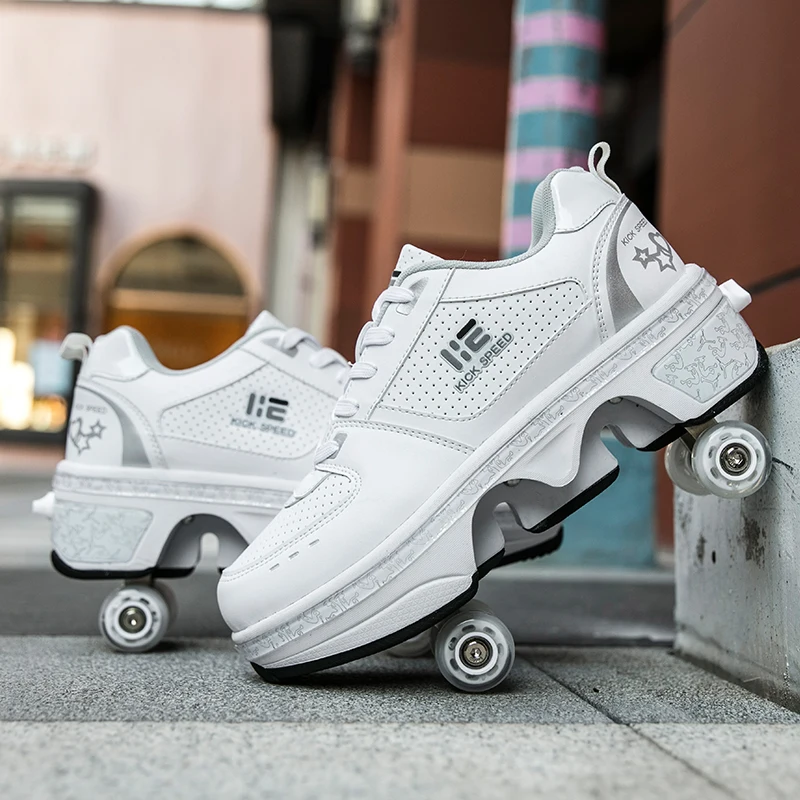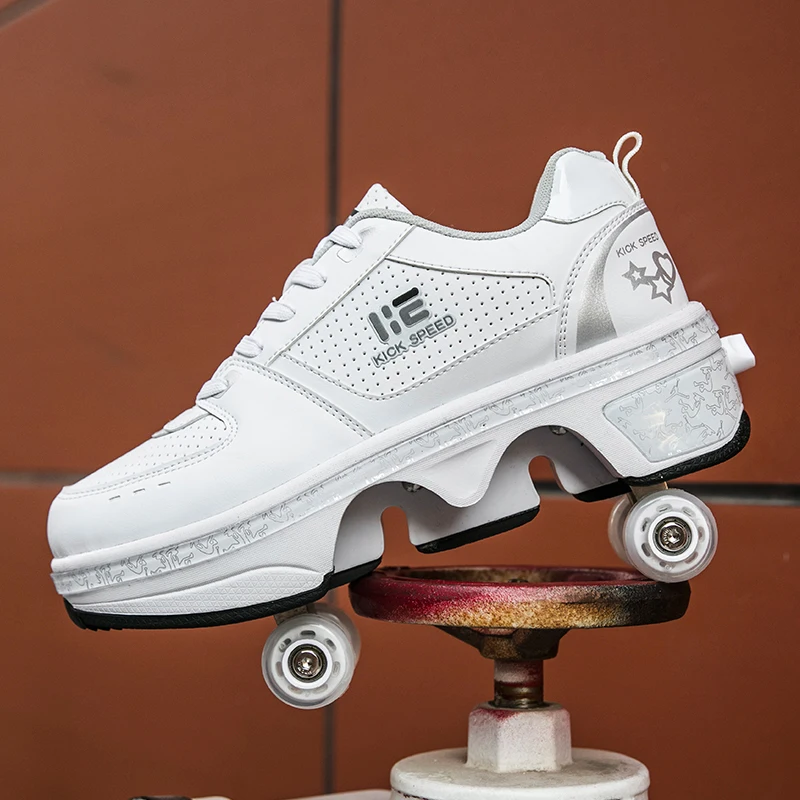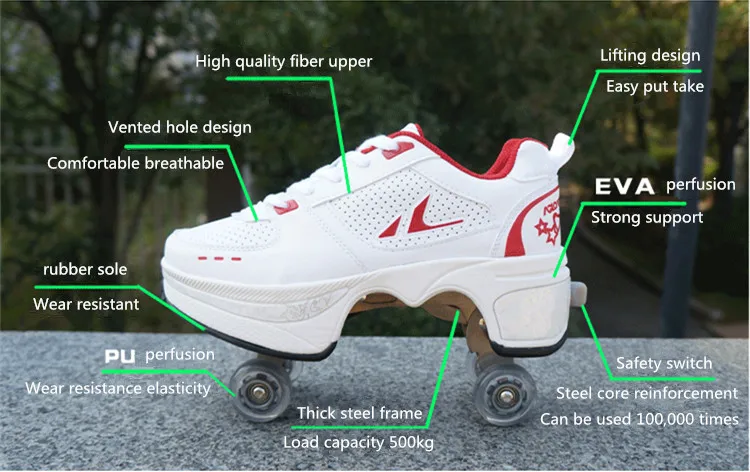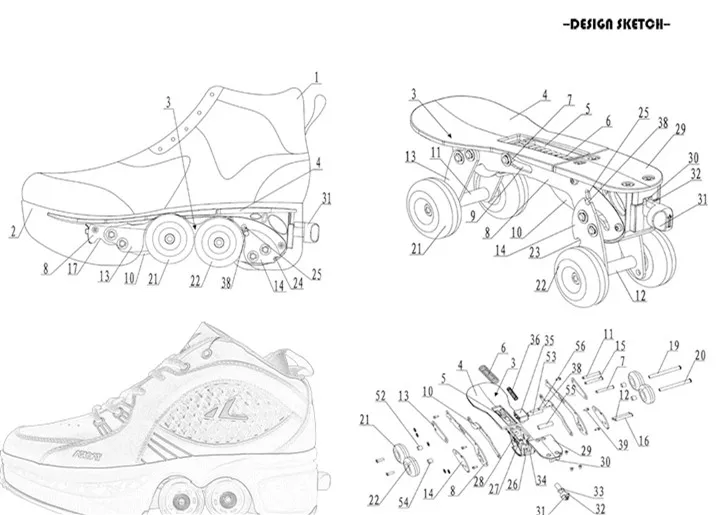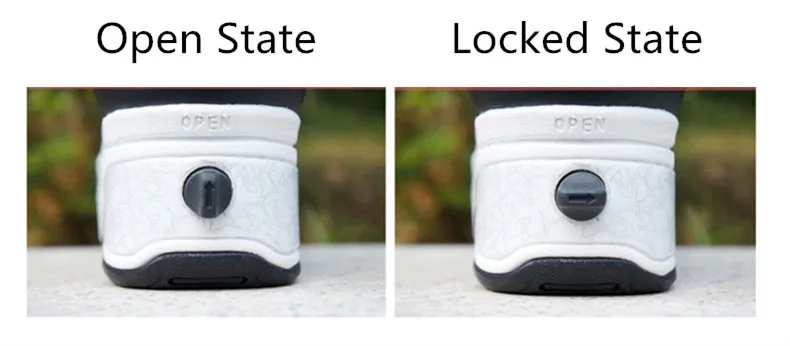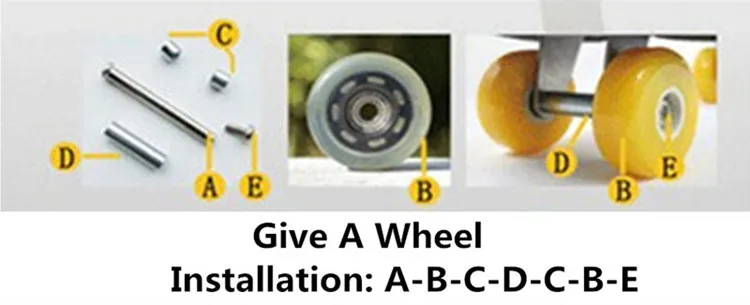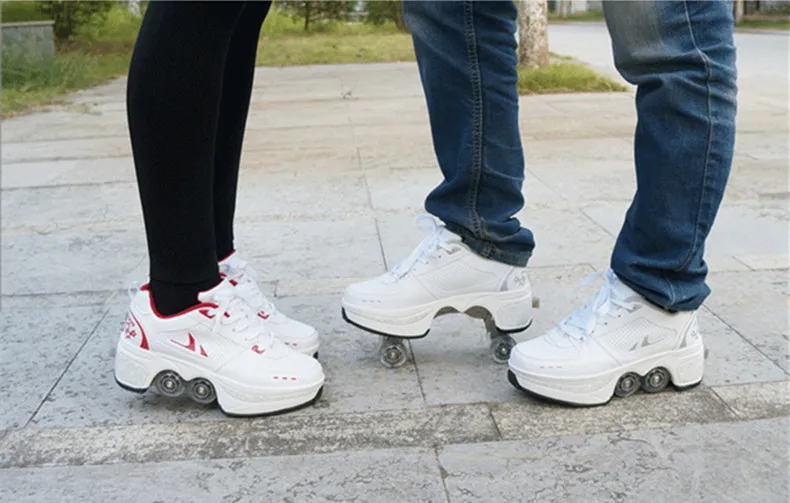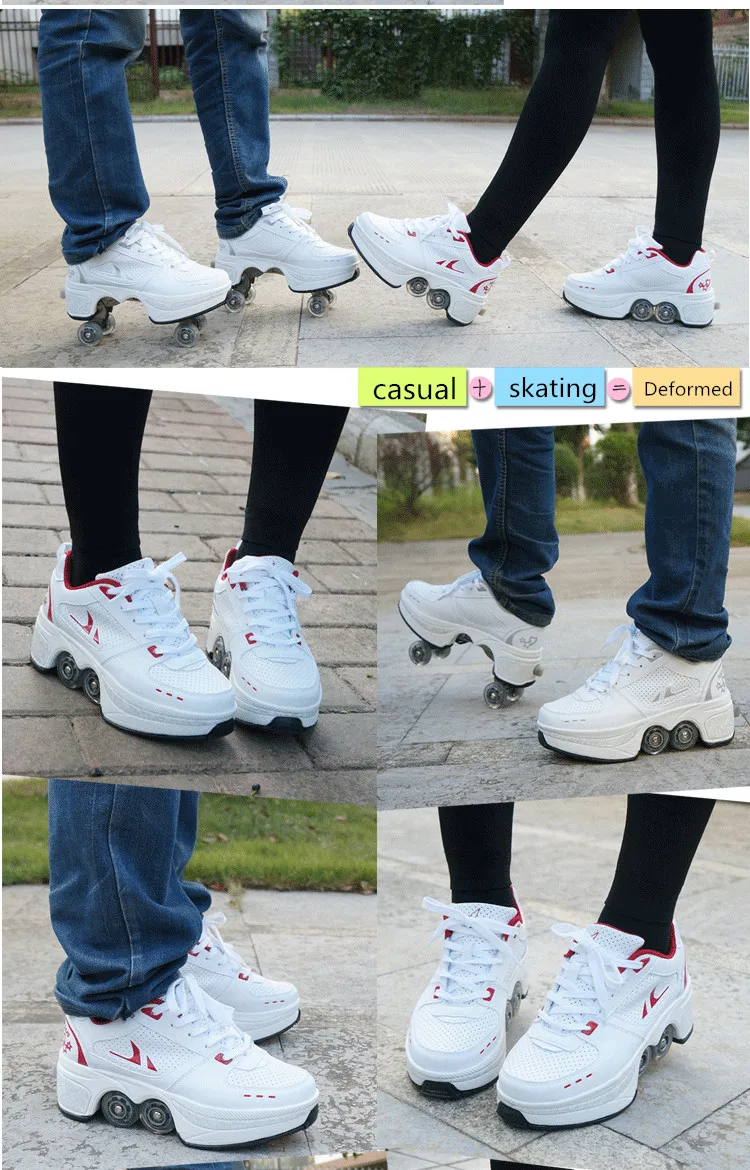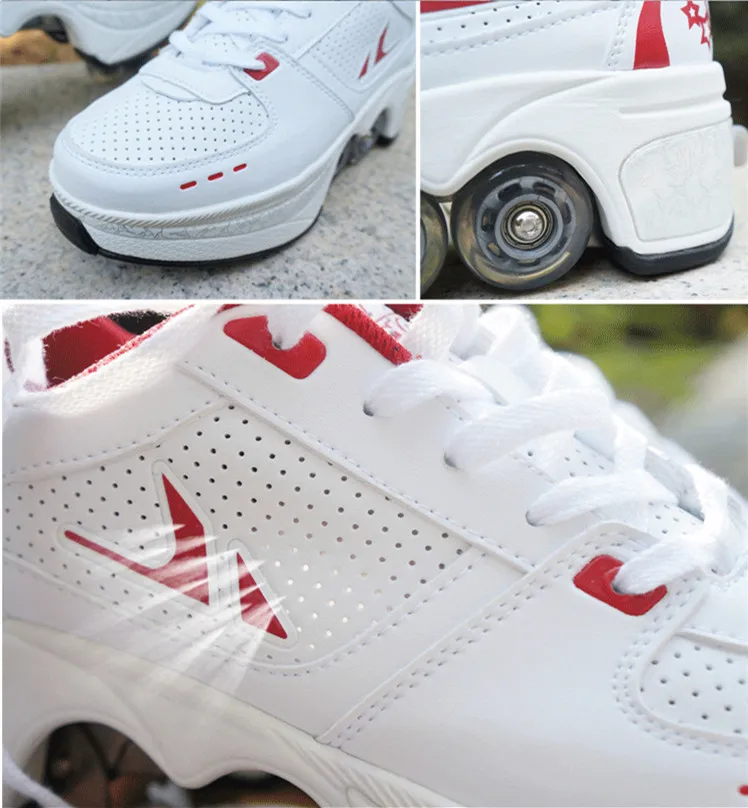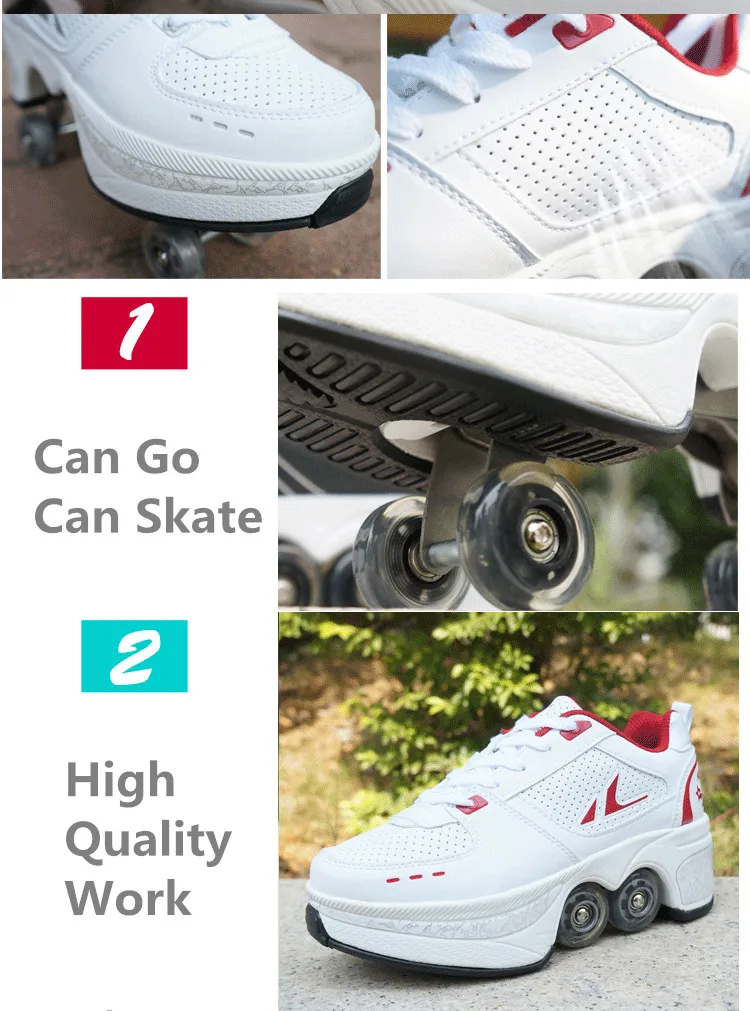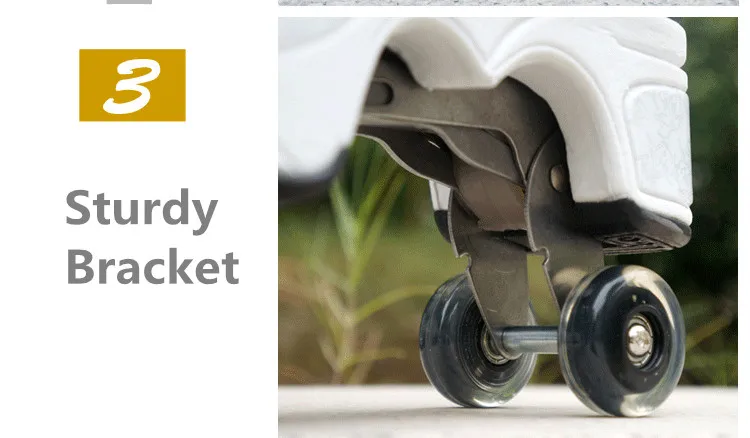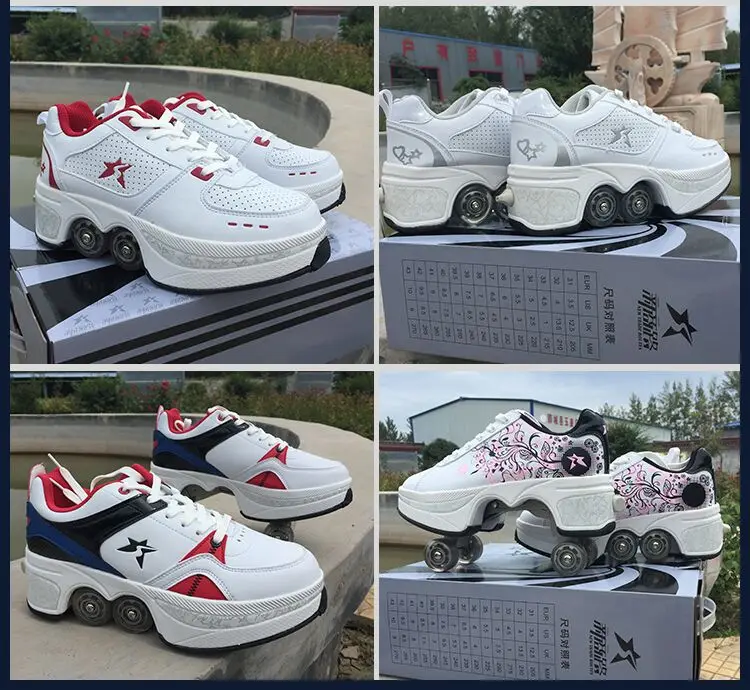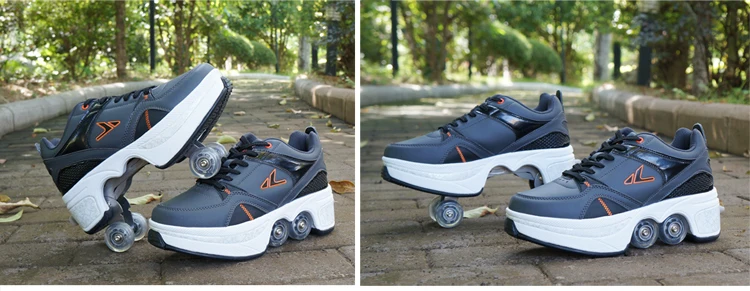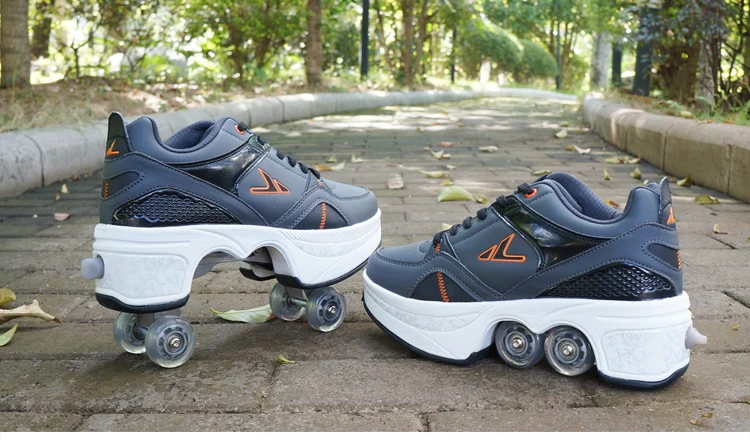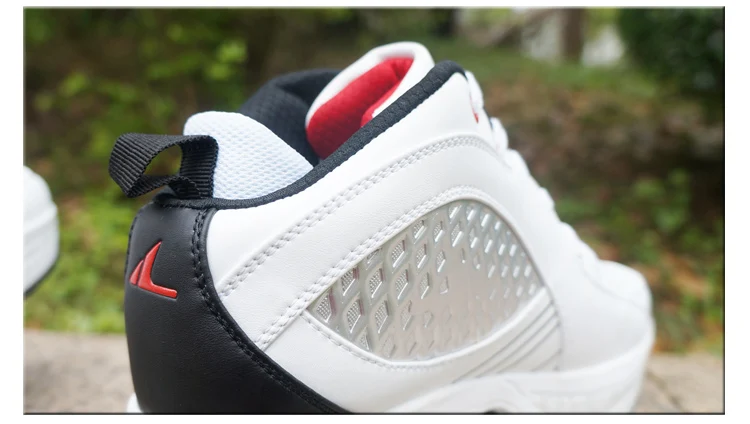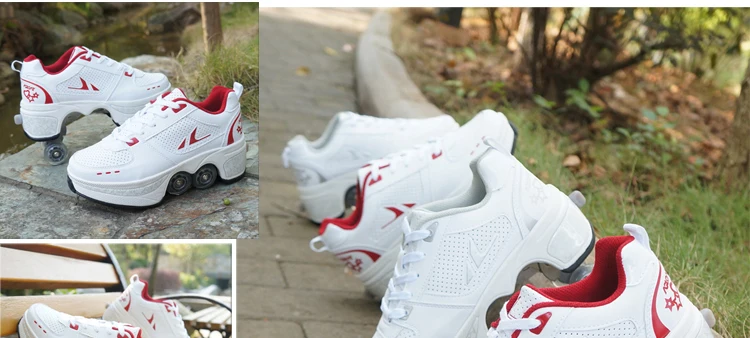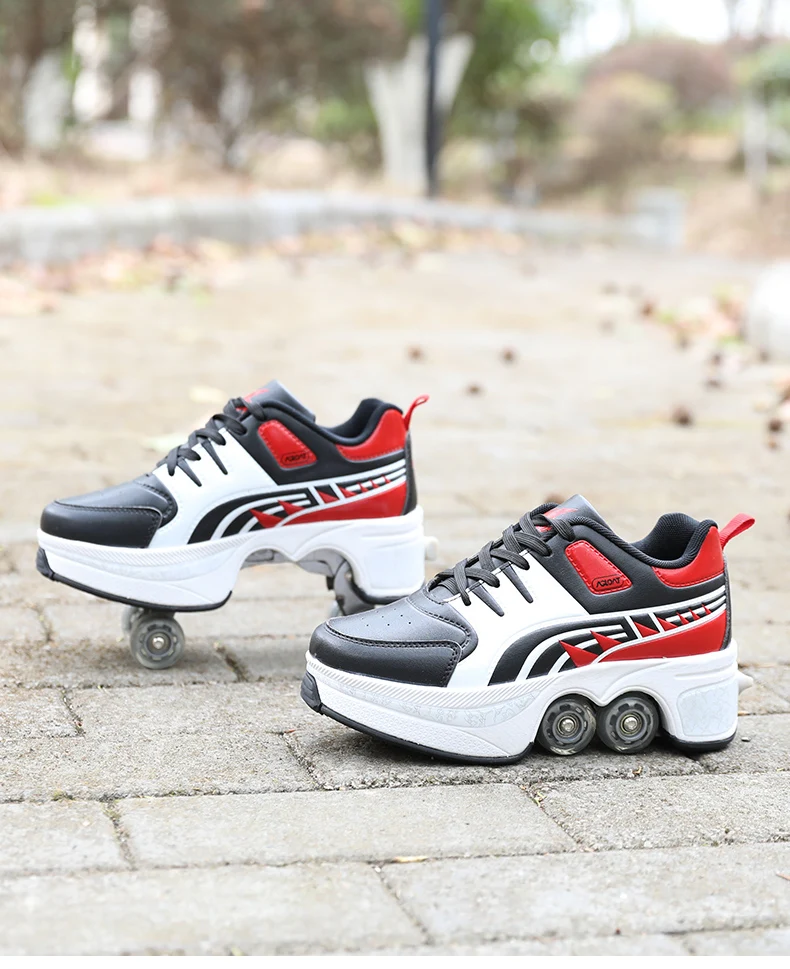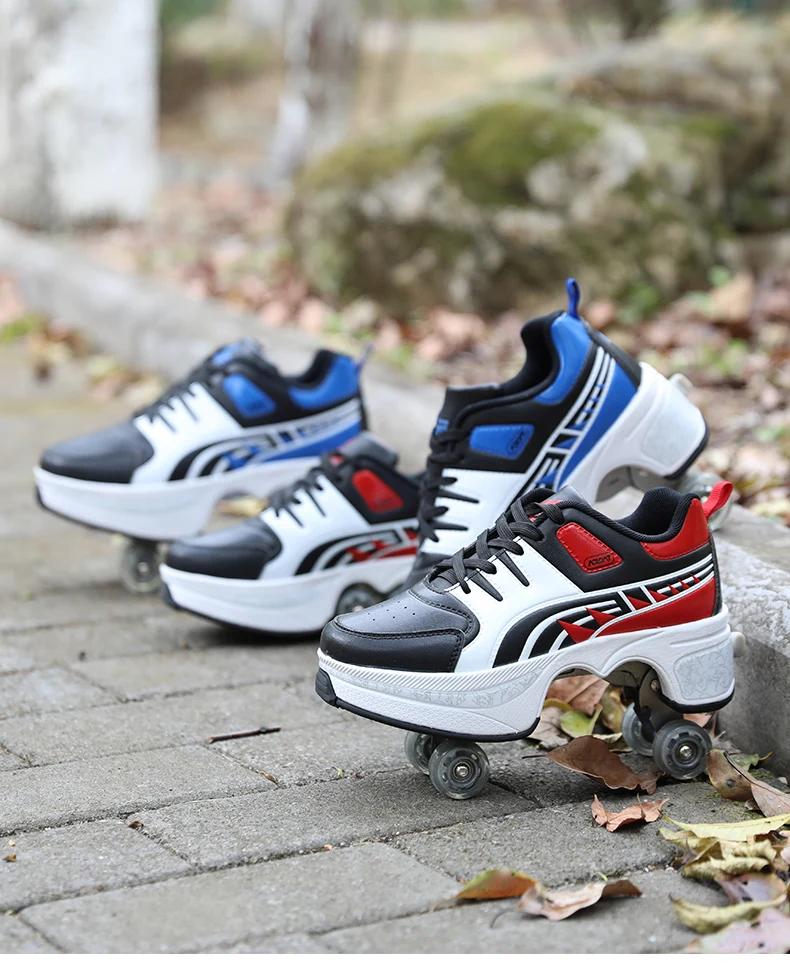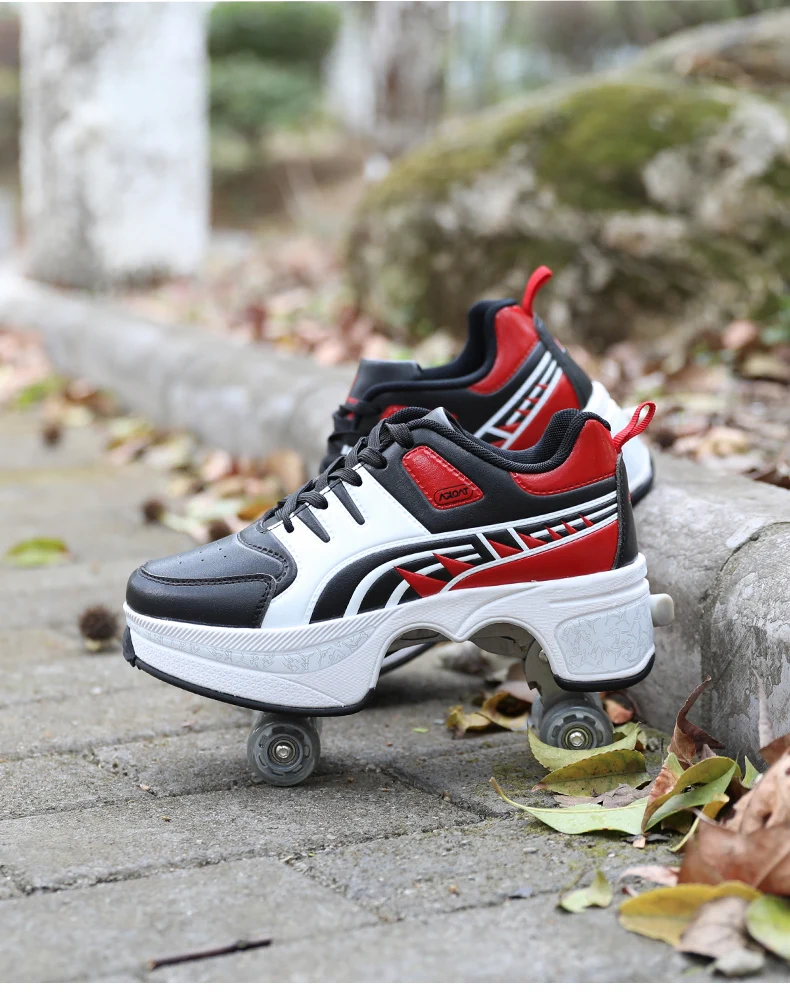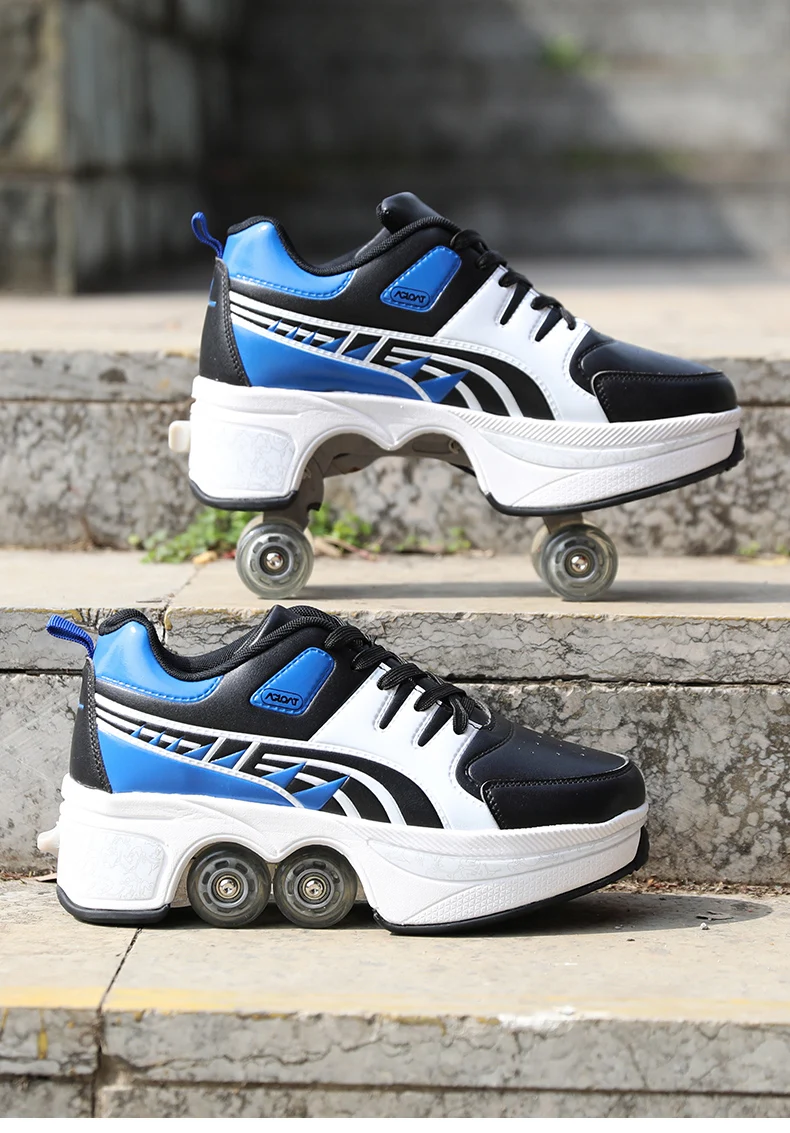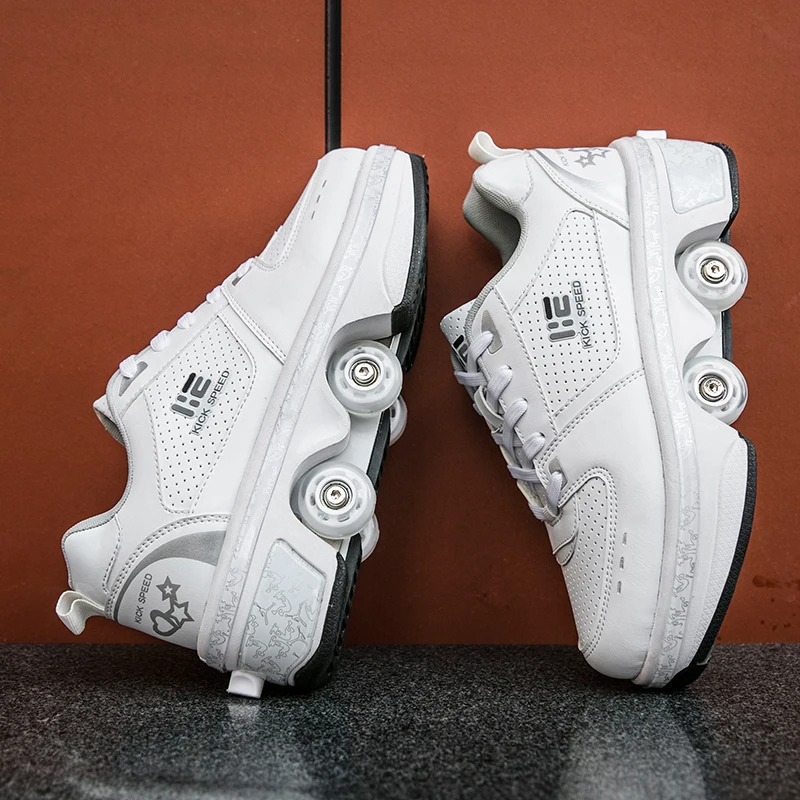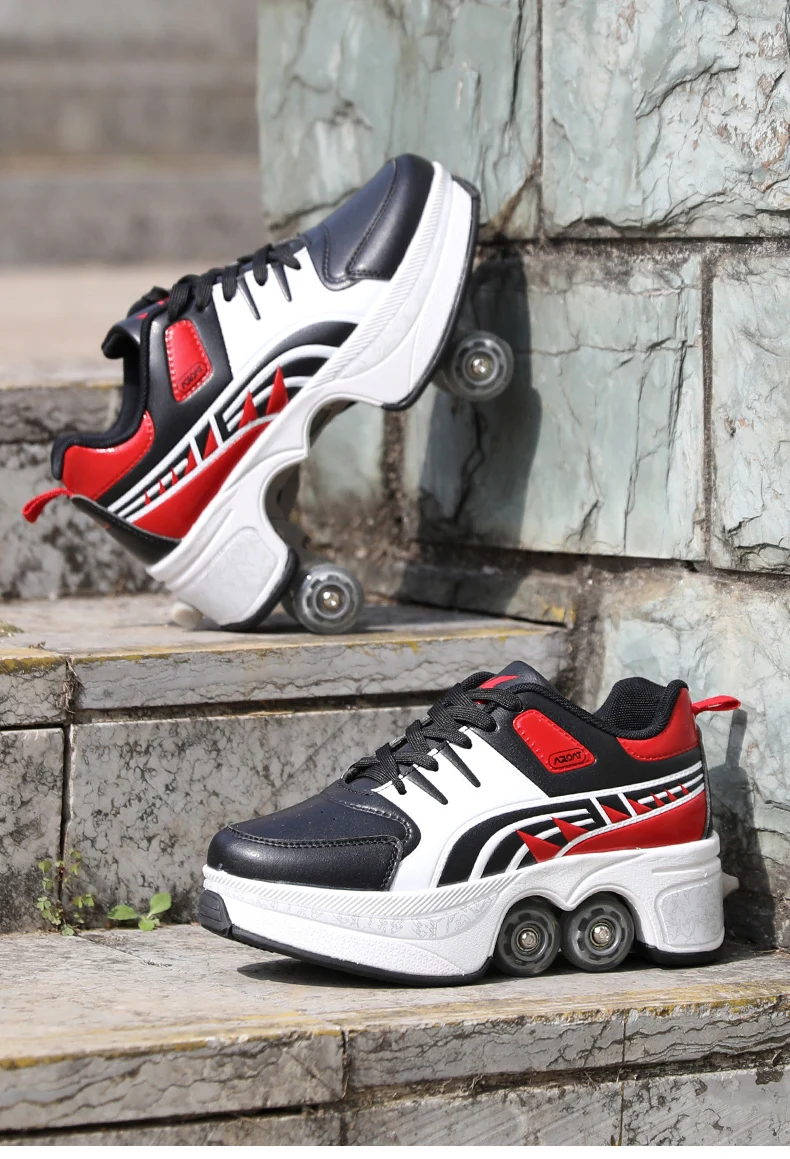Description

SIZE CHART（We are sending European sizes

us size 3.5 = eur size 31 = fit foot lenth 20.5cm

us size 4 = eur size 32 = fit foot lenth 21cm

us size 4.5 = eur size 33 = fit foot lenth 21.5cm

us size 5 = eur size 34 = fit foot lenth 22cm

us size 5.5 = eur size 35 = fit foot lenth 22.5cm

us size 6 = eur size 36 = fit foot lenth 23cm

us size 6.5 = eur size 37 = fit foot lenth 23.5cm

us size 7 = eur size 38 = fit foot lenth 24cm

us size 7.5 = eur size 39 = fit foot lenth 24.5cm

us size 8 = eur size 39.5 = fit foot lenth 25cm

us size 8.5 = eur size 40 = fit foot lenth 25.5cm

us size 9 = eur size 41 = fit foot lenth 26cm

us size 9.5 = eur size 42 = fit foot lenth 26.5cm

us size 10 = eur size 43 = fit foot lenth 27cm